Question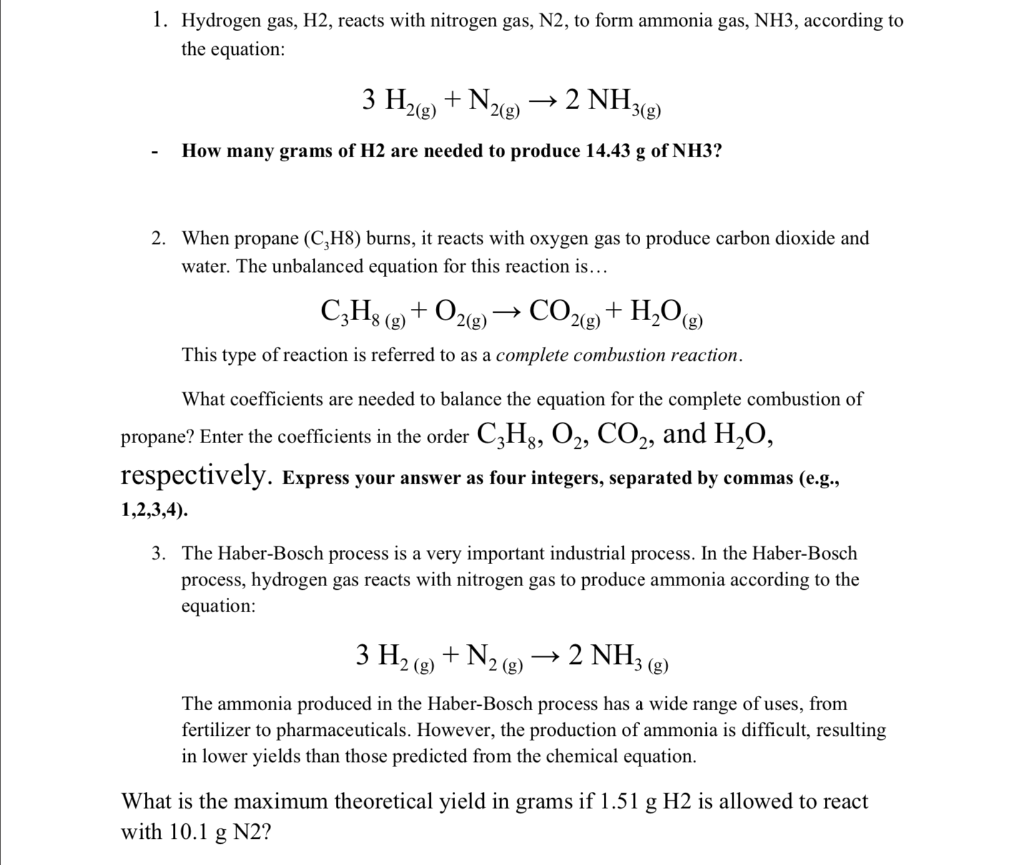1) 2.55g H​​​​​​2 needed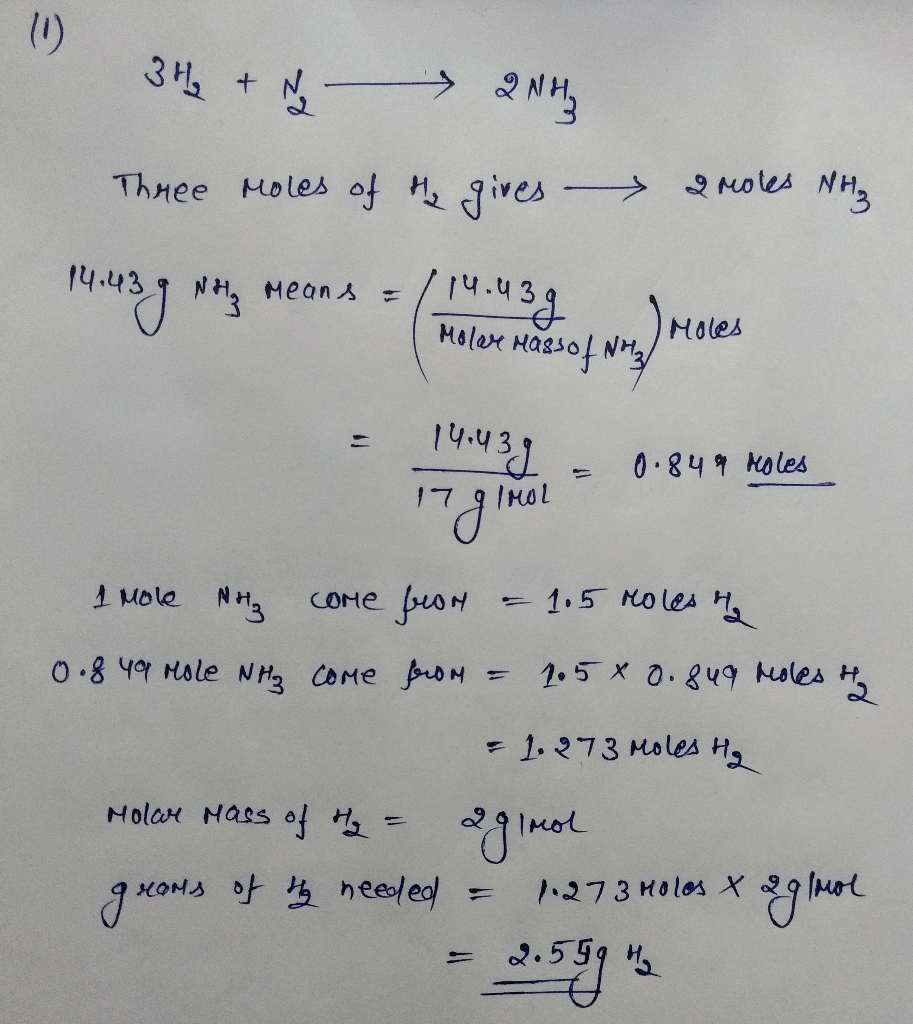2) 1, 5, 3, 4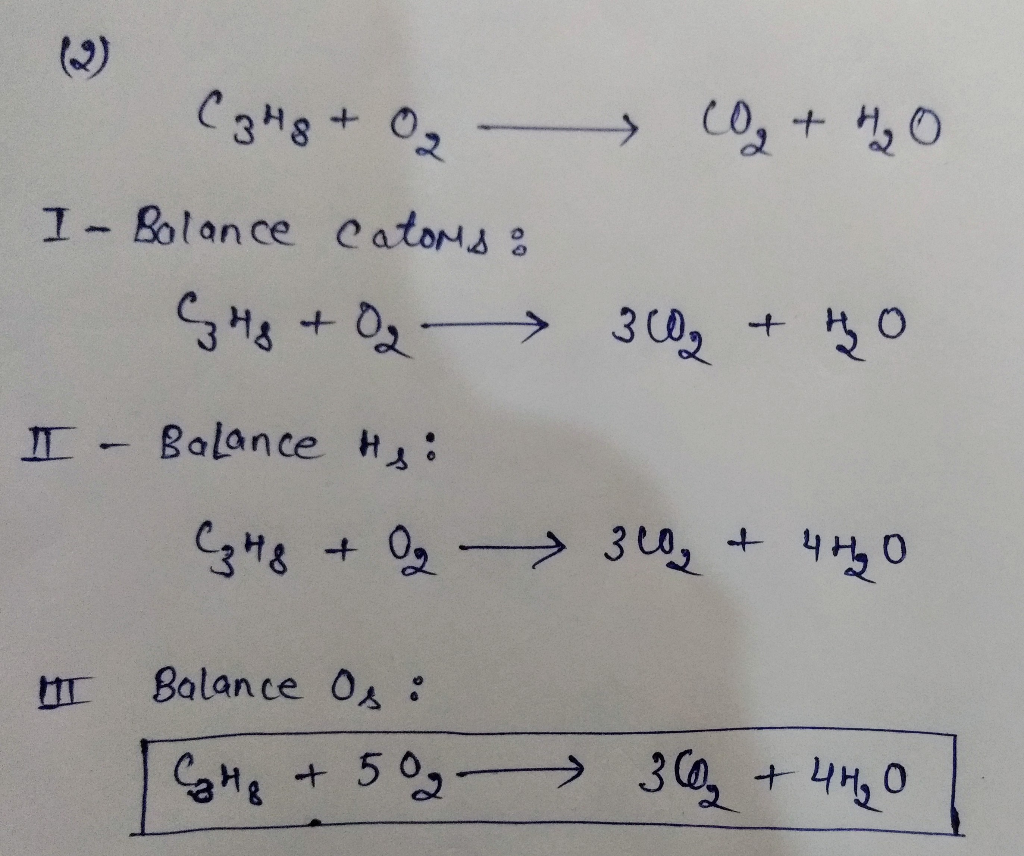#### Earn Coins

Coins can be redeemed for fabulous gifts.

Similar Homework Help Questions
• ### 1. Hydrogen gas, H2, reacts with nitrogen gas, N2, to form ammonia gas, NH3, according to...1. Hydrogen gas, H2, reacts with nitrogen gas, N2, to form ammonia gas, NH3, according to the equation 2 NH3( 3 Нуе) + N, 2(g) 2(g) How many grams of H2 are needed to produce 14.43 g of NH3? 2. When propane (C,H8) burns, it reacts with oxygen gas to produce carbon dioxide and water. The unbalanced equation for this reaction is... С,На (9 + Оzе) CO2(g)H20(g) (g) This type of reaction is referred to as a complete combustion reaction...

• ### The Haber-Bosch process is a very important industrial process. In the Haber-Bosch process, hydrogen gas reacts...

The Haber-Bosch process is a very important industrial process. In the Haber-Bosch process, hydrogen gas reacts with nitrogen gas to produce ammonia according to the equation 3H2(g)+N2(g)→2NH3(g)3H2(g)+N2(g)→2NH3(g) The ammonia produced in the Haber-Bosch process has a wide range of uses, from fertilizer to pharmaceuticals. However, the production of ammonia is difficult, resulting in lower yields than those predicted from the chemical equation. 1.36 g H2 is allowed to react with 9.75 g N2, producing 1.75 g NH3 What is the...

• ### The Haber-Bosch process is a very important industrial process. In the Haber-Bosch process, hydrogen gas reacts...

The Haber-Bosch process is a very important industrial process. In the Haber-Bosch process, hydrogen gas reacts with nitrogen gas to produce ammonia according to the equation 3H2(g)+N2(g)→2NH3(g) The ammonia produced in the Haber-Bosch process has a wide range of uses, from fertilizer to pharmaceuticals. However, the production of ammonia is difficult, resulting in lower yields than those predicted from the chemical equation. 1.43 g H2 is allowed to react with 9.70 g N2, producing 2.31 g NH3. Part A: What...

• ### Hydrogen gas, H2, reacts with nitrogen gas, N2, to form ammonia gas, NH3, according to the...

Hydrogen gas, H2, reacts with nitrogen gas, N2, to form ammonia gas, NH3, according to the equation 3H2(g)+N2(g)→2NH3(g) NOTE: Throughout this tutorial use molar masses expressed to five significant figures. How many molecules (not moles) of NH3 are produced from 4.21×10−4 g of H2?

• ### The Haber-Bosch process is a very important industrial process. In the Haber-Bosch process, hydrogen gas reacts...

The Haber-Bosch process is a very important industrial process. In the Haber-Bosch process, hydrogen gas reacts with nitrogen gas to produce ammonia according to the equation 3H2(g)+N2(g)→2NH3(g) The ammonia produced in the Haber-Bosch process has a wide range of uses, from fertilizer to pharmaceuticals. However, the production of ammonia is difficult, resulting in lower yields than those predicted from the chemical equation. 1.26 g H2 is allowed to react with 9.75 g N2, producing 1.63 g NH3. Part A) What...

• ### The Haber-Bosch process is a very important industrial process. In the Haber-Bosch process, hydrogen gas reacts...

The Haber-Bosch process is a very important industrial process. In the Haber-Bosch process, hydrogen gas reacts with nitrogen gas to produce ammonia according to the equation 3H2(g)+N2(g)→2NH3(g) The ammonia produced in the Haber-Bosch process has a wide range of uses, from fertilizer to pharmaceuticals. However, the production of ammonia is difficult, resulting in lower yields than those predicted from the chemical equation. 1.10 g H2 is allowed to react with 9.72 g N2, producing 1.68 g NH3. Part A What...

• ### The Haber-Bosch process is a very important industrial process. In the Haber-Bosch process, hydrogen gas reacts...

The Haber-Bosch process is a very important industrial process. In the Haber-Bosch process, hydrogen gas reacts with nitrogen gas to produce ammonia according to the equation 3H2(g)+N2(g)→2NH3(g) The ammonia produced in the Haber-Bosch process has a wide range of uses, from fertilizer to pharmaceuticals. However, the production of ammonia is difficult, resulting in lower yields than those predicted from the chemical equation. 1.94 g H2 is allowed to react with 10.1 g N2, producing 1.59 g NH3. Part A What...

• ### In the Haber-Bosch process, nitrogen and hydrogen gas are reacted under high temperature 500 degrees C and pressure 200 atm to form ammonia gas (NH3) according to the following equation: N2+3H2 = 2NH3 If 9300 L H2 gas is reacted with an excess of N2 gas

In the Haber-Bosch process, nitrogen and hydrogen gas are reacted under high temperature 500 degrees C and pressure 200 atm to form ammonia gas (NH3) according to the following equation: N2+3H2 = 2NH3 If 9300 L H2 gas is reacted with an excess of N2 gas at 200 atm and 500 degrees C, how many moles of ammonia gas would be produced, assuming a 15% yield?

• ### 1.13 g H, is allowed to react with 9.69 g N2, producing 2.19 g NH3- The...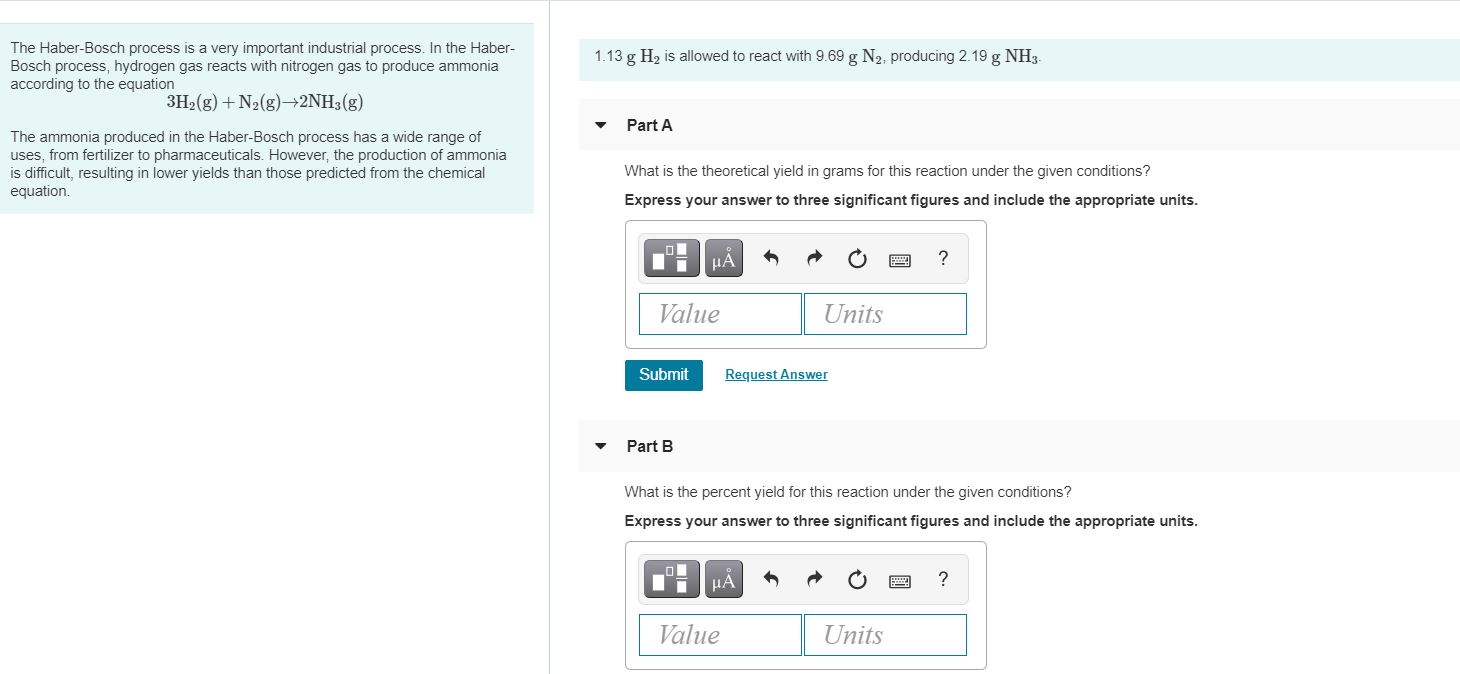1.13 g H, is allowed to react with 9.69 g N2, producing 2.19 g NH3- The Haber-Bosch process is a very important industrial process. In the Haber- Bosch process, hydrogen gas reacts with nitrogen gas to produce ammonia according to the equation 3H2(g) + N2(g)2NH3(g) Part A The ammonia produced in the Haber-Bosch process has a wide range of uses, from fertilizer to pharmaceuticals. However, the production of ammonia is difficult, resulting in lower yields than those predicted from the...

• ### Hydrogen reacts with nitrogen to form ammonia (NH3) according to the reaction 3 H2(g) + N2(g)...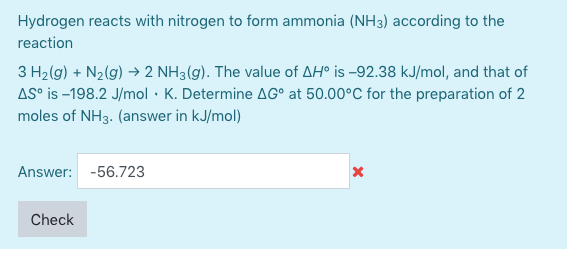Hydrogen reacts with nitrogen to form ammonia (NH3) according to the reaction 3 H2(g) + N2(g) → 2 NH3(g). The value of AH is -92.38 kJ/mol, and that of AS is -198.2 J/mol · K. Determine AG at 50.00°C for the preparation of 2 moles of NH3. (answer in kJ/mol) Answer: -56.723 Check